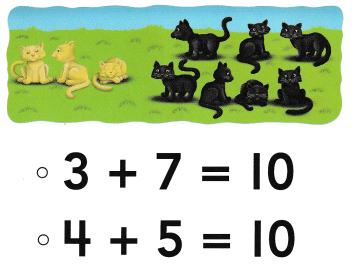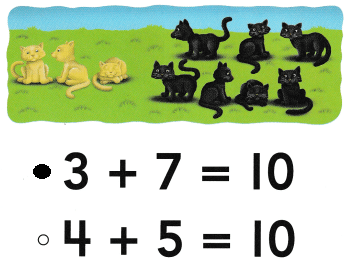# Texas Go Math Kindergarten Lesson 13.4 Answer Key Sums Up to 10

Refer to our Texas Go Math Kindergarten Answer Key Pdf to score good marks in the exams. Test yourself by practicing the problems from Texas Go Math Kindergarten Lesson 13.4 Answer Key Sums Up to 10.

## Texas Go Math Kindergarten Lesson 13.4 Answer Key Sums Up to 10

EssentiaI Question
How can you show and write addition sentences for sums up to 10?

Explore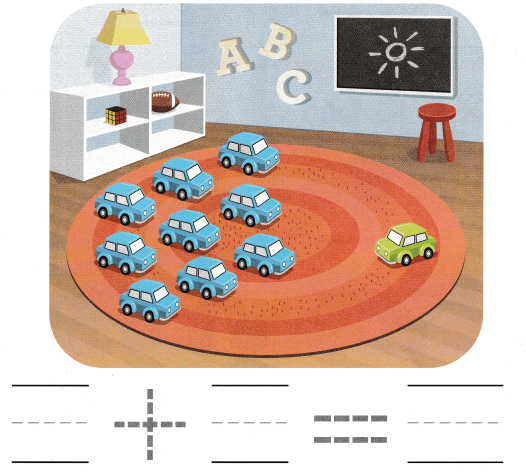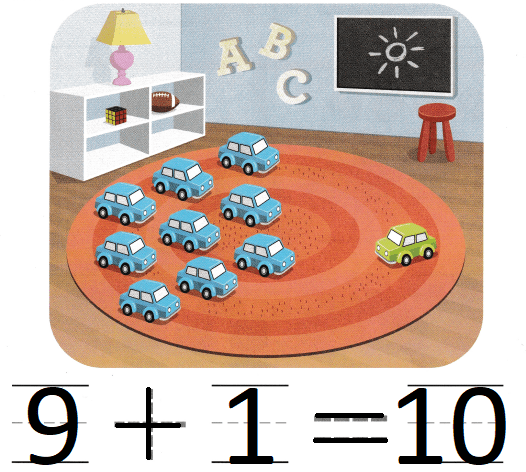Explanation:
9 blue cars and 1 green car
9 + 1 = 10
The sum of 9 and 1 is 10

Directions
Listen to the addition word problem. Write the numbers to complete the addition sentence. Trace the symbols.

Share and Show

Question 1.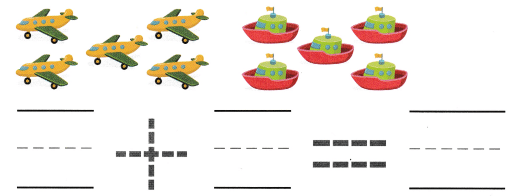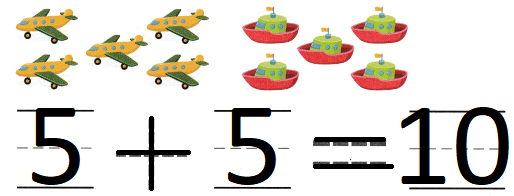Explanation:
5 aero planes and 5 ships
5 + 5 = 10
The sum of 5 and 5 is 10.

Question 2.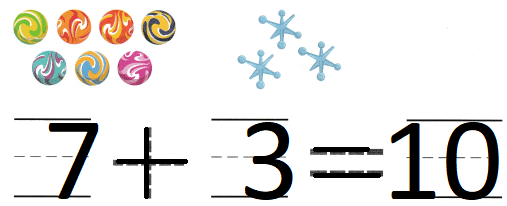Explanation:
7 marbles and 3 stars
7 + 3 = 10
The sum of 7 and 3 is 10

Question 3.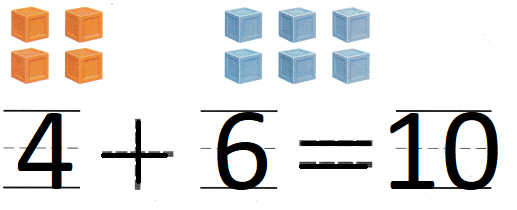Explanation:
4 orange boxes and 6 blue boxes
4 + 6 = 10
The sum of 4 and 6 is 10.

Directions

Question 4.Explanation:
2 fishes and 8 yellow ducks
2 + 8 = 10
The sum of 2 and 8 is 10

Question 5.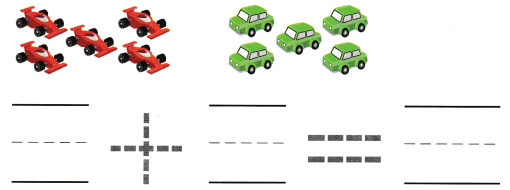Explanation:
5 red cars and 5 green cars
5 + 5 = 10
The sum of 5 and 5 is 10

Question 6.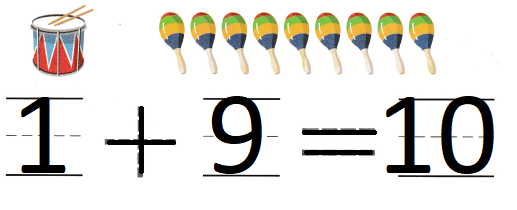Explanation:
1 drum and 9 toys
1 + 9 = 10
The sum of 1 and 9 is 10

Directions

Home Activity

• Have your child tell you an addition sentence with a sum of ten.

Problem Solving

Question 7.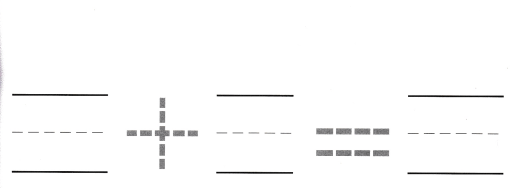Explanation:
There are ten children in the cafeteria.
Ten of them are drinking water.
10 + 0 = 10
0 children are drinking milk

Question 8.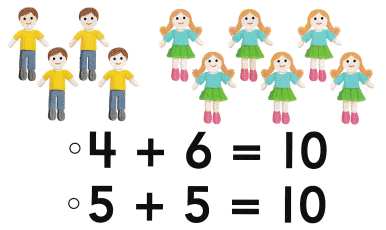Explanation:
Nate has 4 dolls.
Ollie has 6 dolls.
4 + 6 = 10
so, Nate and olive have 10 toys altogether

Directions
7. There are ten children in the cafeteria. Ten of them are drinking water. How many children are drinking milk? Draw counters to show the children drinking milk. Complete the addition sentence. 8. Choose the correct answer. Nate has 4 dolls. Ollie has 6 dolls. What does the picture show?

### Texas Go Math Kindergarten Lesson 13.4 Homework and Practice

Question 1.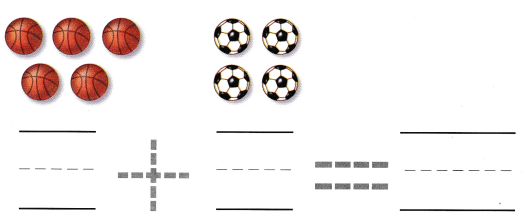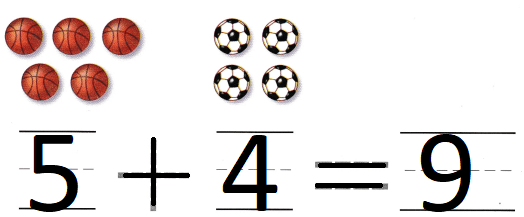Explanation:
She also has 4 soccer balls.
5 + 4 = 9
so, 9 balls that she have in all.

Question 2.Explanation:
The team put seven hats on the rack,
They also put three gloves on the rock.
7 + 3 = 10
10 hats and gloves are on the rack

Directions
Listen to the addition problem. Complete the addition sentence. 1. Kim has five basketballs. She also has 4 soccer balls. How many balls does she have in all? 2. The team put seven hats on the rack, They also put three gloves on the rock. How many hats and gloves are on the rack?

Texas Test Prep

Lesson Check

Question 3.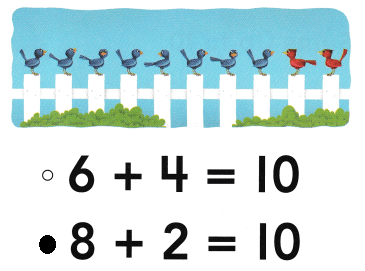Explanation:
here are eight blue birds sitting on a fence.
There are two red birds on the fence.
8 + 2 = 10
so, 10 birds are in the fence.

Question 4.